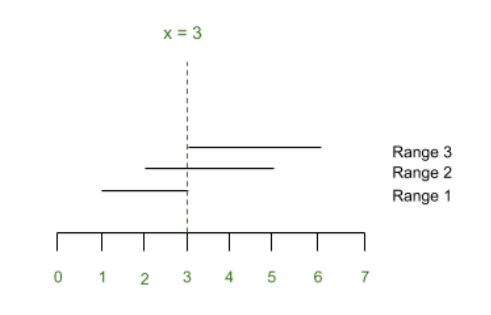# Minimum number of integers required such that each Segment contains at least one of them

Given two arrays start[] and end[] consisting of positive integers denoting the starting and ending points of a segment respectively, the task is to find the minimum number of integers which lies in at least one of the given segments and each segment contains at least one of them.

Examples:

Input: start[] = {1, 2, 3}, end[] = { 3, 5, 6}
Output: 3
Explanation:
All three ranges ([1, 3], [2, 5], [3, 6]) contains the integer 3.

Input: start[] = {4, 1, 2, 5}, end[] = {7, 3, 5, 6}
Output: 3 6
Explanation:
Segments {1, 3} and {2, 5} are contains the integer 3.
Segments {4, 7} and {5, 6} contains the integer 6.

Mathematical formulation:
The mathematical way of describing the problem is to consider each given range of integers to be a line segment defined by two integer coordinates [ai, bi] on a line. Then the minimum number of integers required to cover each of the given range is the minimum number of points such that each segment contains at least one point.
The representation of Example 1 is shown below:Naive approach:
The simplest way to solve the problem is to find the least value of all the starting points and maximum value of all ending points of all segments. Iterate over this range, and for each point in this range keep track of the number of segments which can be covered using this point. Use an array to store the number of segments as:

arr[point] = number of segments that can be covered using this point

1. Find the maximum value in the array arr[].
2. If this maximum value is equal to N, the index corresponding to this value is the point which covers all segments.
3. If this maximum value is less than N, then the index corresponding to this value is a point which covers some segments.
4. Repeat the steps 1 to 3 for array arr[] excluding this maximum value until the sum of all the maximum values found is equal to N.

Time Complexity: O((A-B+1)*N), where A is maximum of ending points of segments and B is the minimum of the starting points of the segments.
Auxiliary Space: O(1)

Efficient Approach:
The problem can be solved efficiently by using the Greedy Technique. Follow the steps given below to solve the problem:

• Sort the segments by their end points.
• Select the point(or coordinate) corresponding to minimum end point of all segments.
• Now, All the segments whose starting point are less than this selected point and whose ending points are greater than this selected point can be covered by this point.
• Then print the minimum number of points.

Below is the implementation of the above approach:

## Python3

 `# python program for the above approach ` ` `  `# Function to compute minimum number ` `# of points which cover all segments ` `def` `minPoints(points): ` ` `  `    ``# Sort the list of tuples by  ` `    ``# their second element. ` `    ``points.sort(key ``=` `lambda` `x: x[``1``]) ` ` `  `    ``# To store the solution ` `    ``cordinates ``=` `[] ` `    ``i ``=` `0` ` `  `    ``# Iterate over all the segments ` `    ``while` `i < n: ` ` `  `        ``seg ``=` `points[i][``1``] ` `        ``cordinates.append(seg) ` `        ``p ``=` `i ``+` `1` ` `  `        ``if` `p >``=` `n: ` `            ``break` ` `  `        ``# Get the start point of next segment ` `        ``arrived ``=` `points[p][``0``] ` ` `  `        ``# Loop over all those segements whose ` `        ``# start point is less than the end ` `        ``# point of current segment ` `        ``while` `seg >``=` `arrived: ` ` `  `            ``p ``+``=` `1` `            ``if` `p >``=` `n: ` `                ``break` `            ``arrived ``=` `points[p][``0``] ` `        ``i ``=` `p ` ` `  `# Print the possibles values of M ` `    ``for` `point ``in` `cordinates: ` `        ``print``(point, end ``=``" "``) ` ` `  ` `  `# Driver Code ` `n ``=` `4`  ` `  `# Starting points of segments ` `start ``=` `[``4``, ``1``, ``2``, ``5``] ` ` `  `# Ending points of segments ` `end ``=` `[``7``, ``3``, ``5``, ``6``]  ` ` `  `points ``=` `[] ` ` `  `# Insert ranges in points[] ` `for` `i ``in` `range``(n): ` `    ``tu ``=` `(start[i], end[i]) ` `    ``points.append(tu) ` ` `  `# Function Call ` `minPoints(points) `

Output:

```3 6
```

Time Complexity: O(N*log N)
Auxiliary Space: O(N)

Attention reader! Don’t stop learning now. Get hold of all the important DSA concepts with the DSA Self Paced Course at a student-friendly price and become industry ready.

My Personal Notes arrow_drop_upCheck out this Author's contributed articles.

If you like GeeksforGeeks and would like to contribute, you can also write an article using contribute.geeksforgeeks.org or mail your article to contribute@geeksforgeeks.org. See your article appearing on the GeeksforGeeks main page and help other Geeks.

Please Improve this article if you find anything incorrect by clicking on the "Improve Article" button below.